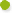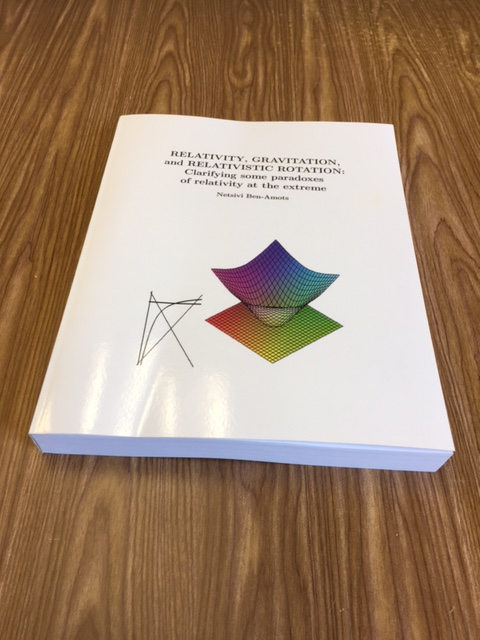﻿ Overview of the book: Relativity, gravitation, and relativistic rotation

Overview

# Relativity, gravitation, and relativistic rotation: clarifying some paradoxes of relativity at the extreme by Netsivi Ben-Amots. Book published 2017 by

Einstein's special relativity says that E=mc2, meaning that m=E/c2. But Einstein defined (1912) for general relativity "...where m denotes a constant that is characteristic of the mass point and independent of the gravitational potential," meaning that the rest mass includes many types of energy, but not the gravitational energy. The book presents how including the gravitational energy solves significant paradoxes.

The second famous equation of special relativity is the relativistic addition of velocities

v1+v2
v = --------------
1+v1v2/c2

When applying this formula to rotation, the obtained formula is not the classical one v=ω r. Philip Franklin (1922) derived a more precise approximate relativistic formula. Using Franklin's relativistic rotation formula instead v=ω r solves additional paradoxes.

Einstein's relativity shows what happens in extreme velocity. This book shows what happens also in extreme accelerations, rotations and other extremities.

In this book the author deals with these questions and others, mainly in simple mathematical terms (integration, differentiation, algebraic equations up to quadratic equations and ordinary differential equations). The author leads the reader through his non-trivial derivations.

A new and surprising world emerges, a world that fits all the present observations and measurements and most of the predictions of general relativity. However, in extreme cases it predicts much more, including a new set of electron orbitals around a proton.

Further, the paradoxes such as black holes, big bang, singularities, dark matter and dark energy disappear without needing anything exotic either.

Also, new explanations to unsolved problems in physics (*) are obtained, including (but not only):How imploding supernovae bounce back outward to enormous explosions,How thick accretion disks accelerate relativistic jets,The structure and stability of quasars and active galactic nuclei and how they produce jets,The sources of energy to these phenomena.

The new solutions presented by the author in this book replace a few paradigms.